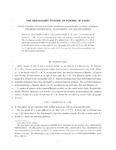## The size-Ramsey number of powers of paths

##### Authors
Clemens, D
Jenssen, M
Kohayakawa, Yhttps://orcid.org/0000-0001-7841-157X
Morrison, N
Mota, GOhttps://orcid.org/0000-0001-9722-1819
##### DOIArticle
##### Abstract

Given graphs G and H and a positive integer q, say that G \emph{is q-Ramsey for} H, denoted G→(H)q, if every q-colouring of the edges of G contains a monochromatic copy of H. The \emph{size-Ramsey number} \sr(H) of a graph H is defined to be \sr(H)=min{|E(G)|:G→(H)2}. Answering a question of Conlon, we prove that, for every fixed~k, we have \sr(Pnk)=O(n), where~Pnk is the kth power of the n-vertex path Pn (i.e., the graph with vertex set V(Pn) and all edges {u,v} such that the distance between u and v in Pn is at most k). Our proof is probabilistic, but can also be made constructive.

2019
2018-12-02
2018-08-18
##### Keywords
powers of paths, Ramsey, size-Ramsey
##### Journal Title
Journal of Graph Theory
0364-9024
1097-0118
91
Wiley